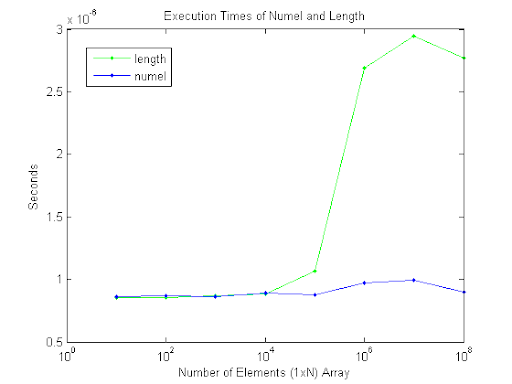# Difference between MATLAB's numel and length functions

I know that `length(x)` returns `max(size(x))` and `numel(x)` returns the total number of elements of x, but which is better for a 1 by n array? Does it matter, or are they interchangeable in this case?

EDIT: Just for kicks:Looks like they're the same performance-wise until you get to 100k elements.

In that case they return the same and there's no difference. In term of performance, it depends on the inner working of arrays in MATLAB. E.g. if there are metainformations about how many elements are in the array (no matter the shape), then numel is as fast as possible, while max(size(x)) seems to need more work to obtain the same thing (retrieving sizes, and then finding the max among those). I am used to use `numel` in that case, but performance speech (hypothetical) apart, I would say they are interchangeable.

• You're right on the performance part. I just ran 100 iterations of numel vs length on x=1:100000000 and numel was on average 3.0919 times faster. Shouldn't matter much for smaller arrays though. – Doresoom Jun 25 '10 at 16:54
• ...and now I'm actually curious about the performance of numel/length vs size of array. – Doresoom Jun 25 '10 at 16:58
• LENGTH will never be the performance bottleneck in your program! Only real performance improvements matter. For all other cases code readability matters. – Mikhail Jun 25 '10 at 17:00
• @Mikhail it depends. First optimization is to write a better algorithm, but avoiding unuseful operations can help to make if faster anyway; of course, no reason to optimize any code that is executed once. (Thanks to Doresoom for testing, I made just a hypotesis about how things could be). I find `numel` readable as `length`, for 1xN arrays (indeed I find `numel` more expressive when usable, but it is subjective) – ShinTakezou Jun 25 '10 at 21:33

For a 1-by-N array, they are essentially the same. For a multidimensional array `M`, they can give different results:

• `numel(M)` is equivalent to `prod(size(M))`.
• `length(M)` is equivalent to `max(size(M))`. If `M` is empty (i.e. any dimension is 0), then `length(M)` is 0.
• Note that if x = ones(0,n); then max(size(x)) --> n while length(x) --> 0 – brown.2179 Feb 11 '15 at 13:23

As other said they are same for one-dimensional array.

IMHO from code readability viewpoint `length` should be used on one-dimensional arrays. It is about "intentional programming", you see the code and understand what programmer had in mind when conceiving his work. So when I see `numel` I know it is used on a matrix.

`length` vs. `numel` was a discussion topic in our team over a number of years. Ex senior developer did not cared about code reability, only about work being done and used only `numel` in otherwise not well readable/formatted code. Other guy is a matematician and used `length` only on numeric arrays being for him "real" arrays. For cell arrays and struct arrays he used `numel`.

• Good perspective - increased readability over a minute/insignificant increase in performance. +1 – Doresoom Jun 25 '10 at 16:56
• When I see NUMEL used, I don't assume it is being used on a matrix versus a vector, I simply assume that it is being used on an object for which the dimensions are unimportant and only the number of elements matters. – gnovice Jun 25 '10 at 17:12
• It matters when you are going to refactor that code and do not know from the first glance whether it is a vector or matrix! – Mikhail Jun 25 '10 at 17:18
• I would argue that it is the variable name that should indicate whether an object is a vector or matrix, not whether NUMEL or LENGTH is used on it. – gnovice Jun 25 '10 at 17:39
• what can I say, I'm that lazy ;) All kidding aside, Even though micro-optimization usually is not worth it, I believe @ShinTakezou has a valid point in that MATLAB's matrices internal structure probably stores the number of elements as meta-information; I came across an interesting but old newsgroup post which studied the `mxArray` structure found in `extern\include\matrix.h`: groups.google.com/group/comp.soft-sys.matlab/browse_thread/… – Amro Jun 25 '10 at 19:27